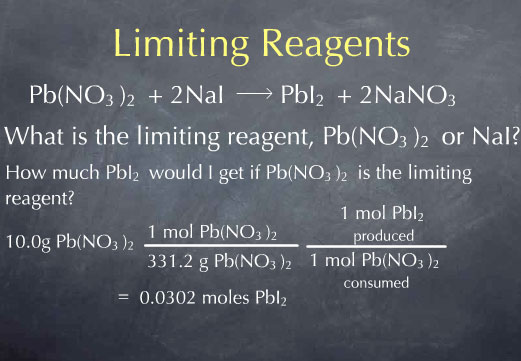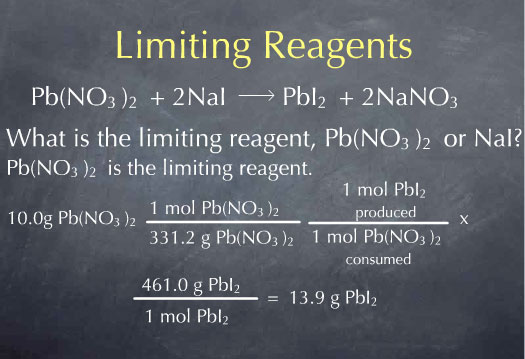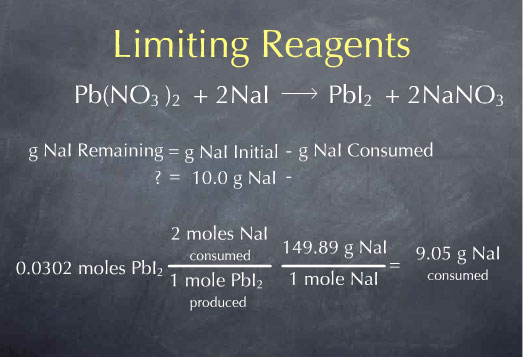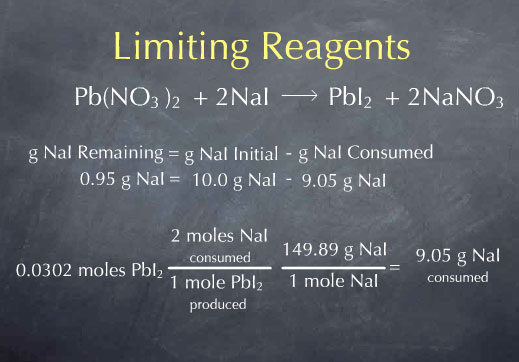# Stoichiometry Tutorials: Limiting Reagents

(from a complete OLI stoichiometry course)

We are now ready to pull everything we know about reaction stoichiometry together, and answer the question: Given some initial amount of reactants, what should be present after a chemical reaction goes to completion? The procedure for answering this question is often called the "limiting reagent problem." You'll see why this name is appropriate in the following video.

### Limiting Reagents Movie Text

Earlier, we were working with the reaction:

Pb(NO3)2 + 2 NaI → PbI2 + 2 NaNO 3

and found that 9.05 g of NaI react completely with 10.0 g of Pb(NO3)2. If we mix 9.05 g of NaI with 10.0 g of Pb(NO3)2, they react completely to form PbI2 and NaNO3. We say that the reactants are in "stoichiometric proportions", meaning that the amount of NaI we are adding is exactly the amount needed to react with the 10.0 g of Pb(NO3)2. Let's say that I'm not being quite so careful, and instead of mixing 9.05 g of NaI with 10.0 g of Pb(NO3)2, I mix 10.0 g of NaI with 10.0 g of Pb(NO3)2. I then ask myself:

What do I have in my solution after this reaction occurs? The reaction is going to continue until something runs out. For every molecule of Pb(NO3)2 that goes away, I'm going to lose two molecules of NaI. So eventually I will run out of either Pb(NO3)2 or NaI . If I mix stoichiometric proportions (9.05 g NaI with 10.0 g Pb(NO3)2), then both compounds are used up at the same time, but in the more general case, I need to first figure out: which reactant runs out first, Pb(NO3)2 or NaI?

We call the reactant that runs out first, the "limiting reagent", so a chemist would rephrase this question as: What is the limiting reagent, Pb(NO3)2 or NaI?

To figure this out, we'll recast it as two separate questions.

1) How much PbI2 would I get if Pb(NO3)2 is the thing that runs out first (if Pb(NO3)2 is the limiting reagent)?

I then ask the same question for the other substance: 2) How much PbI2 would I get if NaI is the thing that runs out first, that is, if NaI is the limiting reagent?

The substance that gives me the smaller amount of product is the one that will run out first. It limits the amount of PbI2 I can form, and so it is the limiting reagent.

I'll begin with the first question. Reactions occur on the molecular scale, so I begin by converting my 10.0 g of Pb(NO3)2to moles of Pb(NO3)2. I can next use the chemical reaction to determine the number of moles of PbI2 produced from this amount of Pb(NO3)2. I do this by multiplying by the ratio: (1 mol of PbI2 produced/ 1 mol of Pb(NO3)2 consumed), and find that 0.0302 moles of PbI2 are produced. This answers my first question.Now, I'll do the same for question 2, regarding NaI. I take my initial 10.0 g of NaI and use the molecular weight to convert from grams to moles. I then look at the chemical reaction and see that for every 2 moles of NaI consumed, 1 mole of lead iodide is produced, which leads to at total 0.0334 moles of PbI2.The answers to these two questions tell me that if I were to consume all the Pb(NO3)2, I would get 0.0302 moles of PbI2, whereas if I were to consume all of the NaI, I would get 0.0334 moles of PbI2. Since 0.0302 is less than 0.0334, I know that the amount of PbI2 produced is limited by the amount of Pb(NO3)2 I had to start with. Therefore, Pb(NO3)2 is the limiting reagent. All of the Pb(NO3)2 is consumed and this gives me 0.0302 moles of PbI2. I can convert this to grams of PbI2 by multiplying by the molecular weight, and I find 13.9 g PbI2 produced by the reaction.I could have guessed from the start that Pb(NO3)2 was the limiting reagent, because I knew it only took 9.05 g of NaI to react with 10.0g of Pb(NO3)2. All of the extra NaI I'm putting in - the difference between 10.0 g and 9.05 g - isn't going to have any Pb(NO3)2 to react with; it's just going to sit there as leftover NaI.

Let's consider a general method we could use to determine the amount of NaI left over after the reaction. The grams of NaI remaining is equal to the grams of NaI I had to start with, minus the grams of NaI consumed. I know that I started with 10.0 g of NaI, so I just need to determine the amount of NaI consumed by the reaction. The difference is the amount of NaI remaining. To do this, I'll start with the amount of PbI2 produced by the reaction, 0.0304 mol PbI2. For every mole of PbI2 produced, I consume 2 moles of NaI, so I can multiply by the ratio: (2 mol NaI consumed/ 1 mol PbI2 produced). I now know the amount of NaI consumed in terms of moles of NaI. I can convert this amount to grams by using the molecular weight. Multiplying by 149.89 g NaI per mole of NaI gives 9.05 g NaI consumed by the reaction.I now know the number of grams NaI consumed. If I subtract this from the grams of NaI I had to start with, I get 0.95 g of NaI remaining.Finding the limiting reagent has helped us achieve our goal of finding out what was left over after the reaction completed.

Use this information to answer the questions below.

The content above has been converted from Adobe Flash Player and may not display correctly.

You may now try a tutor on determining the chemical remediation of arsenic.